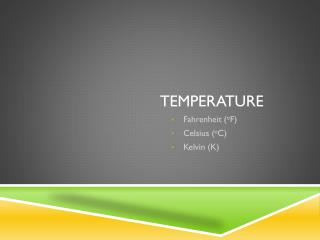DownloadDownload PresentationTemperature

# Temperature

Download Presentation## Temperature

- - - - - - - - - - - - - - - - - - - - - - - - - - - E N D - - - - - - - - - - - - - - - - - - - - - - - - - - -
##### Presentation Transcript

1. Temperature Fahrenheit (oF) Celsius (oC) Kelvin (K)

2. Temperature is… • Is a measure of average energy of motion of the particles of a substance. • The higher the temperature = the faster the particles are moving • The lower the temperature = the slower the particles are moving • Tool for measuring = thermometer

3. Units of measure • Fahrenheit or oF • Celsius or oC • Kelvin or K • All positive numbers • Easier to work with • 0K= absolute zero • The point when all motion stops

4. Conversion Formulas: • Fahrenheit  Celsius • oF= (1.8 x oC) + 32 • Celsius  Fahrenheit • oC= .556 x (oF – 32) • Kelvin  Celsius • K= oC + 273 • Celsius  Kelvin • oC = K - 273

5. Examples • What is 45oF in oC? • Formula= oF = (1.8oC X oC) + 32 • What is 42oC in oF? • Formula= oC= .556 x (oF -32) • What is 60oC in K? • Formula= oC = K – 273 • What is 50K in oC? • Formula= K = oC + 273

6. You Try (show you work!) • Work with your partner, then check with your table • 35oF = _____ oC • 72oC = _____ oF • 345K = _____ oC • 67oC = _____ K • 56oF= ______K • 491K= ________oF

7. Homework • 1.) 72oF oC and K • 2.)